# How can I prove a b c

## ABC formula or midnight formula derivation

The derivation or the proof for the midnight formula (ABC formula) can be found here. We look at this content:

• A Explanationhow to derive the ABC formula or midnight formula.
• A example how to do the derivation.
• Tasks / exercises so that you can practice using the formula.
• A Video to derive the midnight formula / ABC formula.
• A Question and answer area to this topic.

Note: If you are interested in calculating tasks with the formula, please have a look at the midnight formula or the ABC formula.

### Derivation of the midnight formula / ABC formula

How do you actually come up with formulas like the midnight formula? Somebody must have come up with this at least once. How do you get from the quadratic equation / function to the solution equation?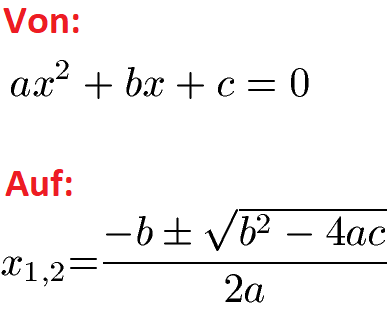A few pointers for a better understanding:

1. There are several ways to derive the ABC formula (midnight formula). For this reason, other sources will have different derivations.
2. We have decided on the path that can be carried out with what we believe to be the least amount of previous knowledge.
3. However, it is not possible without prior knowledge. You should definitely be fit with the application of the binomial formulas, especially the 1st binomial formula. If you don't know these, please learn further under binomial formulas.

All right? Let's look at the derivation for the midnight formula bit by bit.

Display:

### Midnight Formula Proof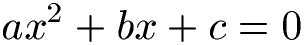We first subtract c. This will give us -c on the right.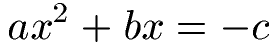We multiply both sides of the equation by 4a. We thereby get: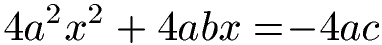We add b on both sides of equation2.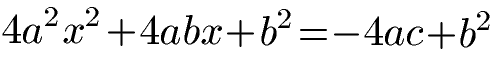Does anyone see anything special in the last equation? Look closely! On the left side is the 1st binomial formula. If you don't remember this any more, you will find it in the next line: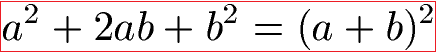You still have no idea about it? In this case, please first take a look at the content under binomial formulas. We apply the 1st binomial formula to the left side.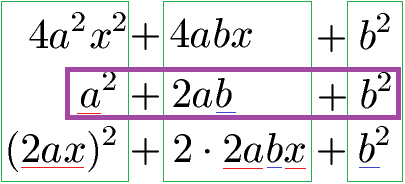As a reminder, on the right side we still have -4ac + b2. We converted the left side: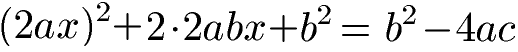We now apply the first binomial formula to the left side and get: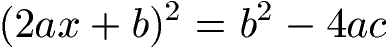We take the roots on both sides of the equation. Note that there is a plus and a minus before the root: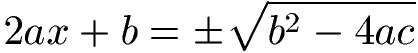We subtract b to the other side: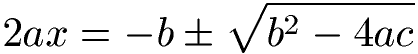They still divide by 2a.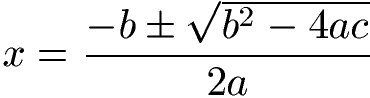Since there are two solutions, it is usually written with x1/2.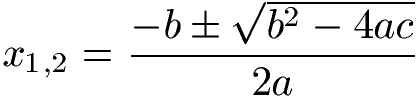Finished!

Show:

### Proof video ABC formula

This video provides a derivation or a proof for the midnight formula or the ABC formula. For a better understanding, an equation is also solved in order to find the corresponding zeros. The next video is from Youtube.com.

Next video »

### Questions with answers midnight formula

In this section, we'll look at typical questions with answers about the Midnight Formula.

Q: What additional content should I watch?
A: Take a look at these topics:

• PQ formula: The PQ formula is an alternative to the ABC formula or midnight formula. But we have an extra article on this. You can find this under the link PQ formula.
• Polynomial division: The polynomial division is also used to find zeros. We also discuss the polynomial division in detail in our article Polynomial division.
• Calculate zeros: The ABC formula or midnight formula is used to find zeros. You can find a complete article on this topic under Calculating Zeros.

Otherwise, of course, the use of the formula derived here under the midnight formula or the ABC formula.

Q: When is the Midnight Formula covered in school?

A: The midnight formula is mostly on the curriculum from 8th grade onwards.